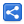# JavaScript - OOP style ES6 class syntax

[Last Updated: Aug 22, 2018]

ES6 (ECMAScript 2015) introduced new OOP style class syntax:

```class Employee {
constructor(name, dept) {
this.name = name;
this.dept = dept;
}
}

let e = new Employee("Sara", "Admin");
console.log(e);```

ES6 class syntax is just a syntactic sugar for existing prototype-based inheritance.

## Getter/Setter

```class Employee {
get name() { return this._name; }
set name(name) { this._name = name; }
get dept() { return this._dept; }
set dept(value) { this._dept = value; }
}

let e = new Employee();
console.log(e);
//using setters
e.name = "Sara";
console.log(e);
//using getter
console.log(e.dept);```

Note that we used underscore for our fields i.e. _name and _dept. That's to avoid function and variable name collision which will end up in recursion like this:

```class X {
set prop(value) { this.prop = value }
}
let x = new X();
x.prop = "something";```

In above example, 'this.prop' calls the same setter method 'set prop()' recursively.

## Class methods

```class Number {
constructor(x) { this.x = x; }
square() { return this.x * this.x; }
}

let number = new Number(4);
console.log(number.square());```

## Static methods

A class can have static methods:

```class Util {
static square(number) {
return number * number;
}
}

let result = Util.square(3);
console.log(result);```

## Static variables

Currently, following is the only way to attach static variables to a class:

```class Util {
static rectangleArea(x, y) {
let area = x * y;
return area.toFixed(Util.DECIMAL_PLACES);
}
}
Util.DECIMAL_PLACES = 2; //static variable

console.log(Util.rectangleArea(5.77, 3.99));```

## Inheritance

The extends keyword can be used to create a subclass.

```class Shape {
constructor(name) { this.name = name; }
draw() { console.log("drawing a shape named " + this.name); }
}

class Circle extends Shape { // creating subclass of Shape
super(name); //calling super class constructor
}

draw() { //overriding super class method
super.draw(); //calling super class draw() method
}
}

let circle = new Circle("circle", 3);
circle.draw();
console.log(circle instanceof Shape);```

## Class Expression

Just like we can create expression for functions:

```let x = function() { console.log("something") }
x();```

We can also create expression for classes:

```let number = class {
constructor(value) { this.value = value; }
square() { return this.value * this.value; }
}
let result = new number(3).square();
console.log(result);```

We can extend another class with a class expression:

```let shapeExpression = class Shape { constructor(name) { this.name = name; } }
let rectangleExpression = class extends shapeExpression {
constructor(name, w, h) {
super(name);
this.w = w;
this.h = h;
}
showArea() { console.log(`Area of \${this.name} is \${this.w * this.h}`); };
}

let rect = new rectangleExpression("square", 7, 7);
rect.showArea();```

## Mix-ins style multiple inheritance

JavaScript only supports single inheritance via 'extends' keyword. We can do mixin style multiple inheritance using class expression (see last example):

```function shape(inputClass) {
return class extends inputClass {
draw() {
console.log("shape is drawing");
}
};
}

function component(inputClass) {
return class extends inputClass {
display() {
console.log("component is displaying");
}
};
}

class Rectangle {
constructor(w, h) {
this.w = w;
this.h = h;
}
area() {
return this.w * this.h;
}
}

let SquareBase = shape(component(Rectangle));
//above is equivalent to: class Shape extends (class Component extends Rectangle{}){}

class Square extends SquareBase {
constructor(sideLength) {
super(sideLength, sideLength); //calling Rectangle constructor
}
}

let square = new Square(4);
square.draw();
square.display();
let area = square.area();
console.log(area);```

 Share